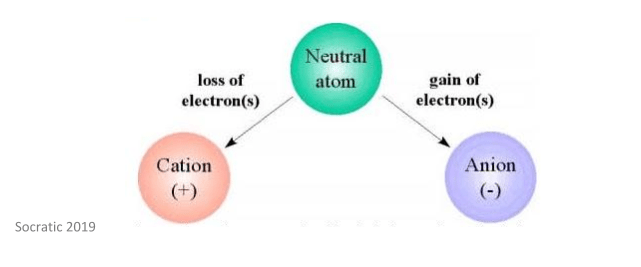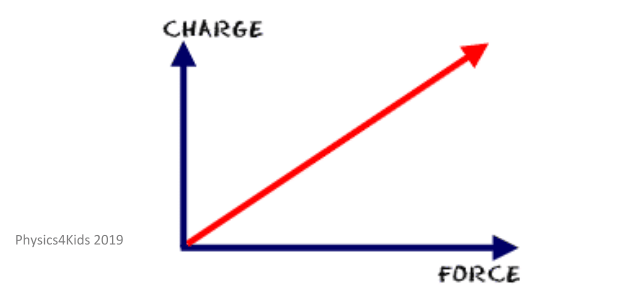Looking for revision notes that are specific to the exam board you are studying? If so, click the links below to view our condensed, easy-to-understand revision notes for each exam board, practice exam question booklets, mindmap visual aids, interactive quizzes, PowerPoint presentations and a library of past papers directly from the exam boards.

# Electric Charge

## Key Information & Summary

• Electric charge is a physical property of matter which causes objects to experience a force when it is placed in an electric field
• Protons carry a positive charge and electrons carry a negative charge
• An ion is an atom that has either gained or lost electrons
• Coulombs are the units of charge
• The total quantity of electric charge is always conserved in an isolated system

## What is electric charge?

In simple terms, electric charge is a physical property of matter – it causes matter to experience a force when it is in an electromagnetic field. There are two types of electric charges:

1. Positive charge which is carried by subatomic particles called protons
2. Negative charge which is carried by subatomic particles called electrons

Neutrons are subatomic particles with no charge. Charges which are the same repel each other, and charges which are different attract each other.

If something contains the same number of protons and electrons, it is said to be neutral overall. Something with more protons has a net positive charge and, as such, something with more electrons has a net negative charge.

## What is an ion?

An ion is an atom that has done one of two things:

• Lost one or more electrons and therefore now has a net positive charge – this is called a cation

Or

• Gained one or more electrons and therefore now has a net negative charge – this is called an anion

This is explained by the image below.As discussed earlier, different charges attract each other. For this reason, cations and anions attract each other to form ionic compounds.

Ions formed of a single atom are called monatomic ions. Ions formed of two or more atoms are called polyatomic ions. There are some special names for certain ions which have multiple charges:

• An ion with a -2 charge is called a dianion
• An ion with a +2 charge is called a dication
• An ion with both positive and negative charges within the same molecule (such as amino acids) are called zwitterions

## Units of charge

The unit of charge is called a coulomb denoted by C. It is most commonly defined as the quantity of charge that passes through the cross-section of an electrical conductor carrying one ampere for one second. 1 coulomb is the quantity of charge in 6.24 x 1018 electrons.

The amount of charge an object has can be measured using a coulombmeter. A nanocoulombmeter can also be used which can measure electrostatic charge down to a nanocoulomb.

## Coulomb’s Law

Coulomb’s law, put simply, states that as the distance increases between two charged objects, the forces and electric fields decrease. Also, the larger the charges on the objects, the larger the forces between them will be. This is shown in the diagram below.This is due to the fact that electric charges form an electric field – this basically shows the direction that a particle with a positive charge will move in when it is placed in the electric field. If two of these fields exist in the same place at the same time (e.g. when a negatively charged particle and a positively charged particle are near each other) they will put force on each other. This is shown well in the image below – you will be able to see how a positively charged particle and a negatively charged particles interact with each other.You need to remember that this law only applies to stationary charges and has been validated for distances up to a few kilometres. Scientists, however, still aren’t sure if this law is valid for distances less than 10-15m.

## Conservation of electric charge

Conservation is the principle that the total quantity of electric charge in an isolated system will never change. This means that the net quantity of charge (so the positive charge minus the negative charges) of the universe will always remain the same – it will be conserved.

Conservation is not the same as saying positive and negative charges cannot be created (and therefore also destroyed). It simply means that in reactions, equal numbers of positive and negative particles are created which therefore helps to keep the net amount of charge the same. The same goes for particles which are destroyed.

As such, it is believed that the net charge of the universe is zero – this means that the quantities of both positive and negative charges in the universe are the same.

## Electric potential

Electric forces and electric fields are both vectors - this means they have both magnitude and a direction. Electric potential only has magnitude.

Electric potential energy is a property of a charged object. If there is a charged object at a specific location, that object is said to have electric potential energy. Put in simpler terms, in order to separate a positive and a negative charge, you have to do ‘work’ – in this process, the charges gain electric potential energy. The amount of ‘work’ needed depends on the amount of charge that has been separated, and how far they have been moved from one another.

Electric potential difference (which can also be called voltage) is the amount of work needed to move a charge from one location to another location within an electric field.